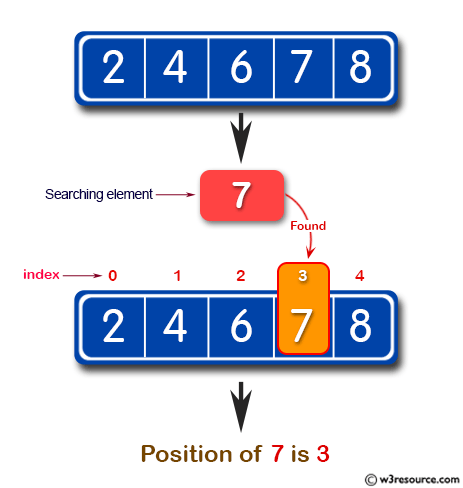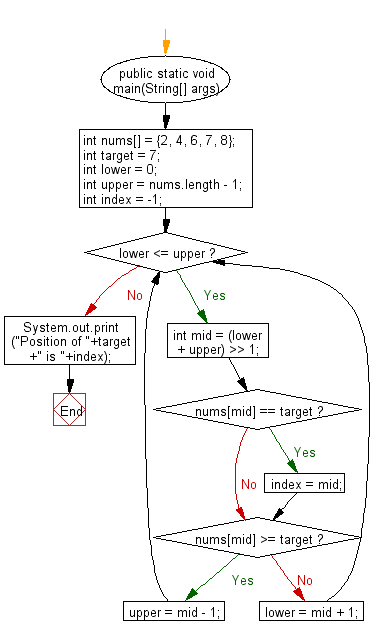﻿ Java: Get the first occurrence of an element of a array# Java Exercises: Get the first occurrence of an element of a given array

## Java Basic: Exercise-119 with Solution

Write a Java program to get the first occurrence (Position starts from 0.) of an element of a given array.

Pictorial Presentation:Sample Solution:

Java Code:

``````import java.util.Scanner;
public class Main {
public static void main(String[] args) {
int nums[] = {2, 4, 6, 7, 8};
int target = 7;
int lower = 0;
int upper = nums.length - 1;
int index = -1;
while (lower <= upper) {
int mid = (lower + upper) >> 1;
if (nums[mid] == target) {
index = mid;
}
if (nums[mid] >= target) {
upper = mid - 1;
} else {
lower = mid + 1;
}
}
System.out.print("Position of "+target +" is "+index);
}
}
```
```

Sample Output:

```Position of 7 is 3
```

Flowchart:Java Code Editor:

What is the difficulty level of this exercise?

Test your Programming skills with w3resource's quiz.

﻿

## Java: Tips of the Day

countOccurrences

Counts the occurrences of a value in an array.

Use Arrays.stream().filter().count() to count total number of values that equals the specified value.

```public static long countOccurrences(int[] numbers, int value) {
return Arrays.stream(numbers)
.filter(number -> number == value)
.count();
}
```

Ref: https://bit.ly/3kCAgLb